New Year, New Start: 15 maths Worksheets That Sharpen Skills

Start the new year off by getting back to basics. Here's a collection of review worksheets that get kids re-acquainted with simple addition and subtraction.

Click on a worksheet in the set below to see more info or download the PDF.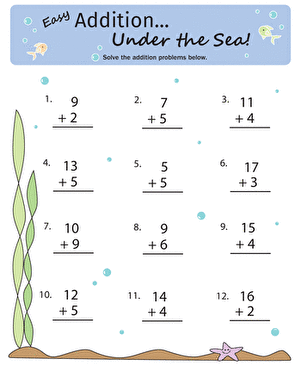Addition Under the Sea

Dive into addition with the single digits in this worksheet, featuring an ocean floor background.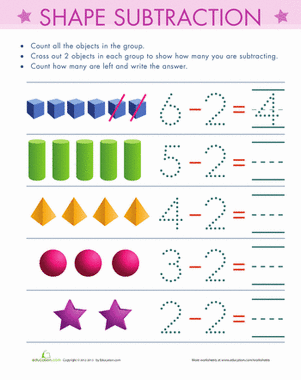Count and Subtract

Ready to introduce your child to subtraction, but you're not sure if he's ready? This worksheet is a simple and easy introduction.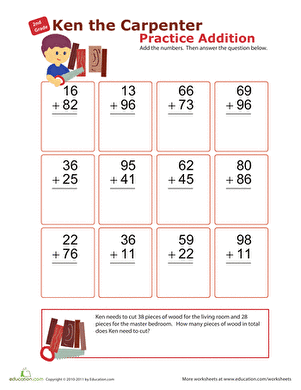Practise Addition with Ken the Carpenter!

Ask your second grader to help out Ken the Carpenter, and his double-digit addition skills will get a boost, too!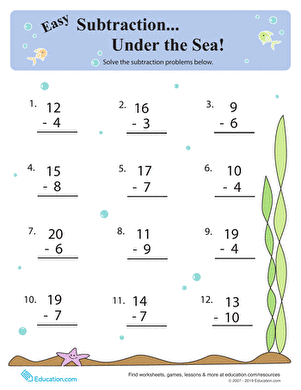Subtraction Under the Sea

Keep your year two maths whiz sharp with this ocean-themed worksheet practising single-digit subtraction.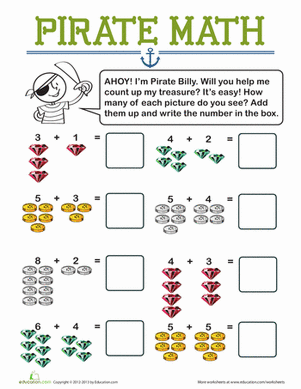Pirate maths

Ahoy! Pirate Billy needs help counting his treasures. Help your kid practise adding single-digit numbers and writing the sums on this treasure-themed worksheet.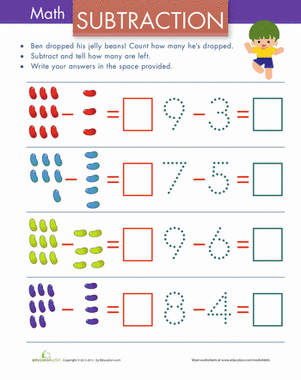Subtraction with Pictures: Jelly Beans

How many jelly beans were dropped? Help your little maths whiz count her way to the difference of each subtraction equation.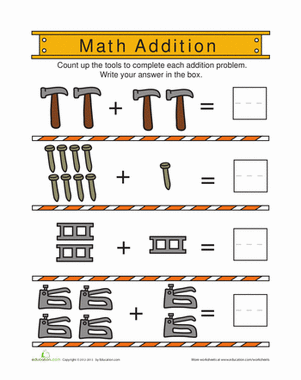Little builders, get ready for some construction zone maths! Your child will use his counting skills to solve these addition equations.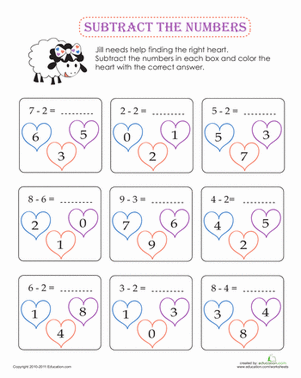Subtraction Coloring

Help Jill the Sheep track down the right heart by completing the subtraction problems in each box.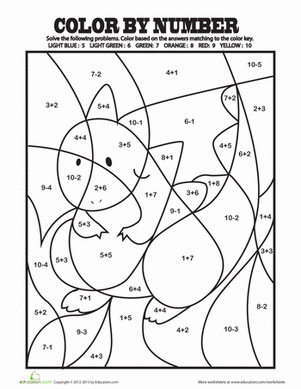Colour by Addition Facts

Challenge your child with some basic addition and subtraction problems. Then she can use the answers to colour by number her own work of art!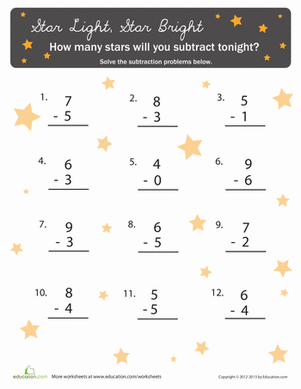Subtracting with Stars

Need to add some sparkle to your child's subtraction practise? Let the stars guide your maths whiz as he tackles 12 problems amidst the stars.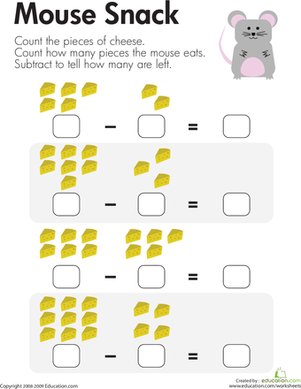Counting Up Subtraction

For each problem on this year two maths worksheet, kids subtract single-digit numbers to see how many cheese pieces are left after the mouse eats its snack.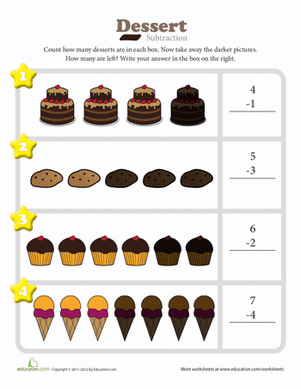Dessert Subtraction

Count the number of desserts in each box then take away the ones that are darker. How many are left?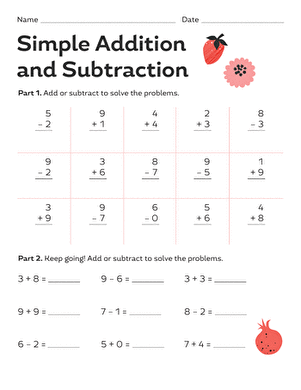Simple Addition and Subtraction

Lend your first grader a hand with beginning maths. She'll practise both addition and subtraction with both vertical and horizontal equations.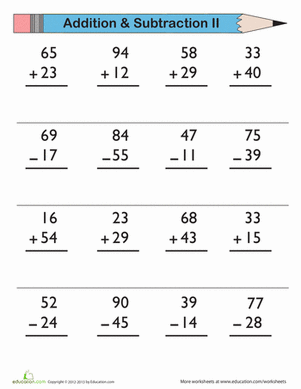Keep those maths skills sharp with a little practise. All you have to do is add and subtract these double-digit numbers.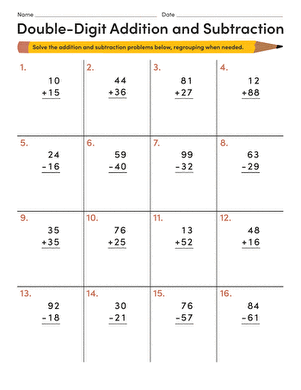Double-Digit Addition and Subtraction

Practise makes perfect! Use this worksheet for a quick practise session in double-digit addition and subtraction for your 1st grader.

Want to download this whole set as a single PDF?

Create new collection

0

New Collection>

0Items

What could we do to improve Education.com?

Please note: Use the Contact Us link at the bottom of our website for account-specific questions or issues.

What would make you love Education.com?